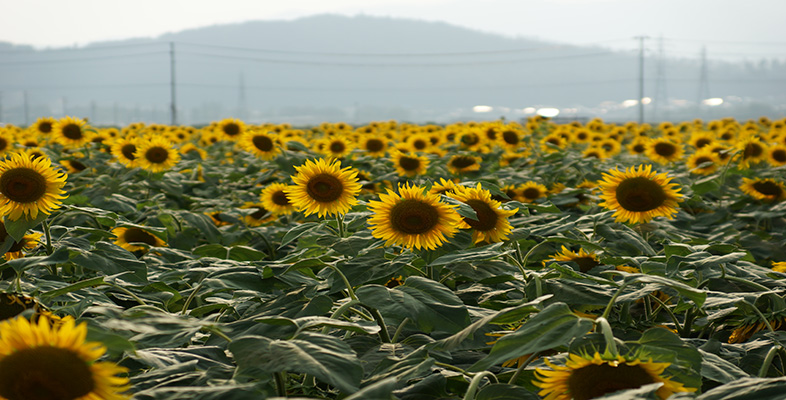Ratio, proportion and percentages

This free course is available to start right now. Review the full course description and key learning outcomes and create an account and enrol if you want a free statement of participation.

Free course

1.2 Expressing ratios

To make short crust pastry, one recipe book says ‘use one part of fat to two parts of flour’; another recipe says ‘use fat and flour in the ratio of one to two’; and yet another says ‘use half as much fat as flour’. These are different ways of expressing the same ratio. Ratios are often expressed as fractions. So in this case: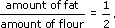Since you can multiply top and bottom of a fraction by the same number and get an equivalent fraction, you can use the ratio in a number of ways. If you have 100 grams of fat then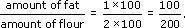So you need 200 grams of flour to 100 grams of fat. There are many ways to arrive at this answer. The important point is that a ratio of 100 to 200 is equivalent to 1 to 2.

To make concrete, the instructions are ‘use sand and cement in the ratio three to one’. This means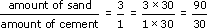If you have 30 kg of cement, then you need 90 kg of sand.

The conversion rates between currencies or different units are often easier to remember as ratios. Many people remember that the ratio of distance in miles to the same distance in kilometres is five to eight.

Example 1

At the time of writing the ratio of prices in pounds sterling to prices in euros is two to three (2 : 3). What is the equivalent price in pounds for a coat costing 150 euros?

3 euros are equivalent to 2 pounds. This means that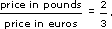So price in pounds = 2/3 × price in euros = 2/3 × 150 = 100

The equivalent price of the coat is £100.

MU120_4M3